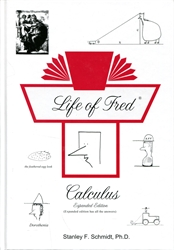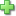Life of Fred: Calculus (Expanded Edition)

(as serious as it needs to be)

Life of Fred
by Stanley F. Schmidt
Hardcover, 591 pages
Price: \$57.00

Calculus, Expanded Edition is the first book in the Life of Fred University Mathematics Series, and covers two years' worth of college-level calculus.

Twenty-four chapters are included; each ends with six problem sets (20-30 minutes each) that are named after individual cities. Worked solutions for all cities problems are provided to students in this expanded edition. Interspersed throughout are instructions to complete the "Your Turn to Play" problems that are grouped together at the back of the book. These are randomly assigned numbers to prevent accidentally seeing an answer while checking answers (e.g. numbered as 45, 888, 500, 317, 923, 71, etc.); all answers are provided. The "Further Ado" appendix provides even more practice opportunities with extra-hard questions for motivated students.

Life of Fred Calculus covers: Functions, Limits, Speed, Slope, Derivatives, Concavity, Trig, Related Rates, Curvature, Integrals, Area, Work, Centroids, Logs, Conics, Infinite Series, Solids of Revolution, Polar Coordinates, Hyperbolic Trig, Vectors, Partial Derivatives, Double Integrals, Vector Calculus, Differential Equations.

• Functions
• Limits
• Speed
• Slope
• Derivatives
• Concavity
• Trig
• Related Rates
• Curvature
• Integrals
• Area
• Work
• Centroids
• Logs
• Conics
• Infinite Series
• Solids of Revolution
• Polar Coordinates
• Hyperbolic Trig
• Vectors
• Partial Derivatives
• Double Integrals
• Vector Calculus
• Differential Equations.
Did you find this review helpful?
Series Description
Related CategoriesClick here to write a review